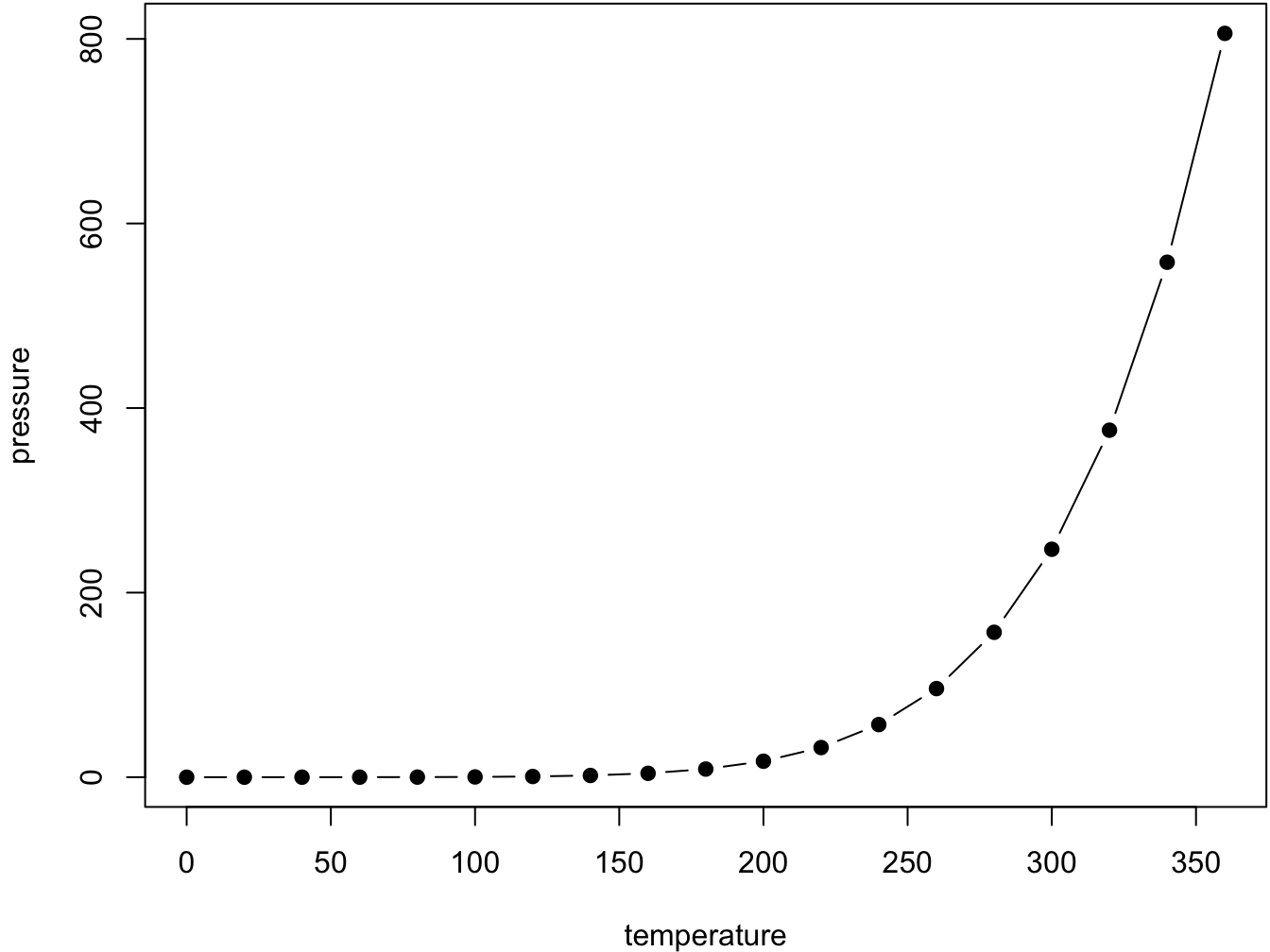## 1.1 Book-wide references

With R Markdown, it is only possible to reference items inside the same document, and there is no numbered figures, tables or equations (at least if LaTeX code is not used, but then, you can only generate a PDF). Bookdown fills the gap with new formatting instructions that work across all documents of the book. It also provides updated versions of R MArkdown output formats that support numbered figures, tables and equations, e.g., html_document2 to replace html_document, for instance.

### 1.1.1 Reference to chapter and titles

You can reference chapters and titles using 1. The book is organized in chapters and all numbers are by chapters. However, there are special level 1 title you can use:

• Parts: use # (PART) Part I {-} just before the first title of that part.

• Appendix: use # (APPENDIX) Appendix {-} just before appendices titles. They will be numbered separately.

### 1.1.2 Numbered figures and tables

Figures and tables are in their own environments:

par(mar = c(4, 4, .1, .1))
plot(pressure, type = 'b', pch = 19)Figure 1.1: Here is a nice figure!

Reference a figure by its code chunk label with the fig: prefix, e.g., see Figure 1.1. Similarly, you can reference tables generated from knitr::kable(), e.g., see Table 1.1.

knitr::kable(
head(iris, 20), caption = 'Here is a nice table!',
booktabs = TRUE
)
Table 1.1: Here is a nice table!
Sepal.Length Sepal.Width Petal.Length Petal.Width Species
5.1 3.5 1.4 0.2 setosa
4.9 3.0 1.4 0.2 setosa
4.7 3.2 1.3 0.2 setosa
4.6 3.1 1.5 0.2 setosa
5.0 3.6 1.4 0.2 setosa
5.4 3.9 1.7 0.4 setosa
4.6 3.4 1.4 0.3 setosa
5.0 3.4 1.5 0.2 setosa
4.4 2.9 1.4 0.2 setosa
4.9 3.1 1.5 0.1 setosa
5.4 3.7 1.5 0.2 setosa
4.8 3.4 1.6 0.2 setosa
4.8 3.0 1.4 0.1 setosa
4.3 3.0 1.1 0.1 setosa
5.8 4.0 1.2 0.2 setosa
5.7 4.4 1.5 0.4 setosa
5.4 3.9 1.3 0.4 setosa
5.1 3.5 1.4 0.3 setosa
5.7 3.8 1.7 0.3 setosa
5.1 3.8 1.5 0.3 setosa

### 1.1.3 Numbered equations

To number equations and allow to refer to them, use an equation environment and label them with the syntax (\#eq:label):

$\begin{equation} f\left(k\right) = \binom{n}{k} p^k\left(1-p\right)^{n-k} \tag{1.1} \end{equation}$

… and here, I refer to eq. (1.1). In the vase equations are not labelled, use the equation* environment instead.